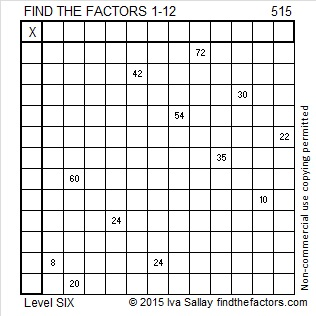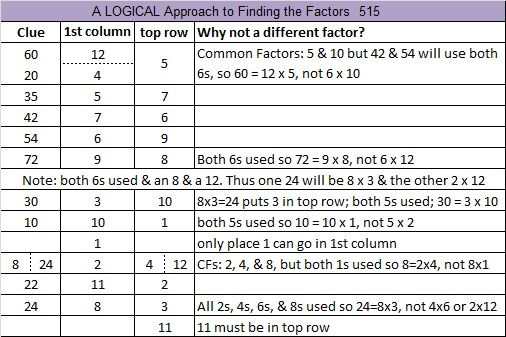# 515 and Level 6

515 = 41 + 43 + 47 + 53 + 59 + 61 + 67 + 71 + 73 which are nine consecutive prime numbers.

515 is the hypotenuse of the Pythagorean triple 309-412-515. What is the greatest common factor of those three numbers?Print the puzzles or type the solution on this excel file: 12 Factors 2015-06-01

—————————————————————————————————

• 515 is a composite number.
• Prime factorization: 515 = 5 x 103
• The exponents in the prime factorization are 1 and 1. Adding one to each and multiplying we get (1 + 1)(1 + 1) = 2 x 2 = 4. Therefore 515 has exactly 4 factors.
• Factors of 515: 1, 5, 103, 515
• Factor pairs: 515 = 1 x 515 or 5 x 103
• 515 has no square factors that allow its square root to be simplified. √515 ≈ 22.6936114—————————————————————————————————This site uses Akismet to reduce spam. Learn how your comment data is processed.